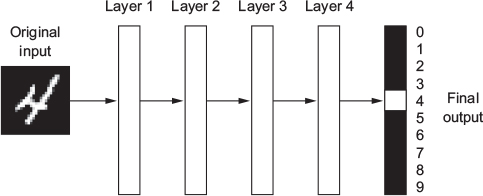## 15.5 The ‘deep’ in deep learning (2)

• What do the representations learned by a deep-learning algorithm look like?
• Let’s examine how a network several layers deep (see Figure 15.2) transforms an image of a digit in order to recognize what digit it is.
• Graph below: visualizes how an image showing “4” is transformed into the number 4Figure 15.2: A deep neural network for digit classification (Chollet & Allaire, 2018, Fig. 1.5)